• 对a数组元素的非法引用
千次阅读
2021-05-22 16:35:19

C语言

第三章 数组

一、选择题

1．合法的数组定义是

A．int a[ ]=”string”; B．int a={0,1,2,3,4,5};

C．char a=”string”; D．char a[ ]={0,1,2,3,4,5};

2．若有定义和语句：char s; s=”abcd”; printf(“%s\n”,s); 则结果是(以下u代表空格)

A．输出abcd B．输出a

C．输出abcd u u u u u D．编译不通过

3．数组a的元素排列次序是

A．a,a,a,a B．a,a,a,a

C．a,a,a,a D．a,a,a,a

4．有以下语句，则下面是正确的描述

static char x [ ]=“12345”; static char y[ ]={ 1 , 2 , 3 , 4 , 5 };

A．x数组和y数组的长度相同。

B．x数组长度大于y数组长度。

C．x数组长度小于y数组长度

D．x数组等价于y数组。

5．列不能正确进行字符串赋值操作的语句是

A．char str;gets(str); B．char*str;str=“a”;

C．char*str;str= a ; D．charstr;strcpy(str,“hello”);

6．若数组的长度为n，则该数组中的元素最多有n个。若是一个二维数组，则最多可使用的元素个数为

A．8 B．10 C．15 D．5

7．若有说明：int a; 则对a数组元素的非法引用是

A．a['B'-'A'][2*1] B．a C．a[4-2] D．a

8．设有语句static char str［10］={“china”};printf(“%d”,strlen(str)); 则输出结果是

A．10 B．5 C．china D．6

9．表达式strlen(“string”)+strlen(“C”)的运算结果为

A．9 B．10 C．7 D．8

10．执行以下程序段后，s的值为。

static char ch[ ]=“623”

int a,s=0;for(a=0;ch[a]>= 0 &&ch[a]<= 9 ;a++)

s=10*s+ch[a]- 0 ;

A．-4705 B．623 C．600 D．326

11．C语言中，数组名代表

A．数组全部元素的值

B．数组首地址 D．数组元素的个数 C．数组第一个元素的值

二、阅读程序，写出运行结果：

1．main()

更多相关内容
• 通过赋初值定义二维数组的大小 第一种： 例如：int a[] = {{1, 2, 3}, {4, 5}, {6}, {8}}; 注意： 对于二维数组，省略只能省略第一个方括号中的常量表达式 如上的初赋值语句等同于： int a = {{1...

通过赋初值定义二维数组的大小

第一种：

例如：int a[] = {{1, 2, 3}, {4, 5}, {6}, {8}};

注意：

对于二维数组，省略只能省略第一个方括号中的常量表达式

如上的初赋值语句等同于：

int a = {{1, 2, 3}, {4, 5}, {6}, {8}};

第二种：int = c[] = {1, 2, 3, 4, 5};

要计算第一个空的方括号中的值x：

当花括号对中的个数能整除第二维的常量表达式时，整数的结果就是第一维的大小；否则，第一维的大小等于所得的结果加1。

（即5/3 = 1 ……2， x = 1 +1 =2）

二维数组和指针

例如：int *p, a;

//定义p为指针变量，a是含有12个元素的一维数组

二维数组实际是一个一维数组，而每一个元素又是一个一维数组。

如上述a，有三个元素a，a，a；而每个元素又是由四个整数元素组成的一维数组。

通过a，a引用，可以把a看做一个整体，当做一个数组名。

a就是代表a的地址。

p = a是合法的        p = a+1 相当于&a

从基类型来看，a为具有4个整型元素的数组类型。

注意：p = a;        //非法    p基类型只有4个字节，a基类型有4*4个字节

二维数组取地址

有变量i和j，它们的取值范围为0 <= I < 3 、0 <= j < 4

那么a[i][j]的地址可以用以下五种表达形式：

（1）&a[i][j]

（2）a[i]+j

（3）*(a+i)+j

（4）&a+4*i+j

（5）a+4*i+j

展开全文指针
• 题目 若有说明int a,则a数组元素非法引用是() A.a B.a C.a[2*1] D.a[4-2] 答案是B,请问c选项呢
• 数组变量属引用类型，数组也可以看成是对象，数组中的每个元素相当于该对象的成员变量。 数组中的元素可以是任何数据类型，包括基本类型和引用类型。 2 一维数组的声明 一维数组的声明方式：type var[]或type[] var ...

1 数组的概念

• 数组可以看成是多个相同类型数据组合，对这些数据的统一管理。
• 数组变量属引用类型，数组也可以看成是对象，数组中的每个元素相当于该对象的成员变量。
• 数组中的元素可以是任何数据类型，包括基本类型和引用类型。

2 一维数组的声明

• 一维数组的声明方式：type var[]type[] var
例如：int a1[]; 　　int[] a2;
double　b[];
Person[] p1; String s1[];
• Java语言中声明数组时不能指定其长度（数组中元素的个数），例如：int a;//非法
• Java中使用关键字new 创建数组对象，格式为：数组名 = new 数组元素的类型[数组元素的个数]
    public class Test {
public static void main(String[] args){
int[] s;
s = new int;
for (int i=0; i < 5;i++){
s[i] = i;
}
}
}
• 元素为引用数据类型的数组中的每一个元素都需要实例化。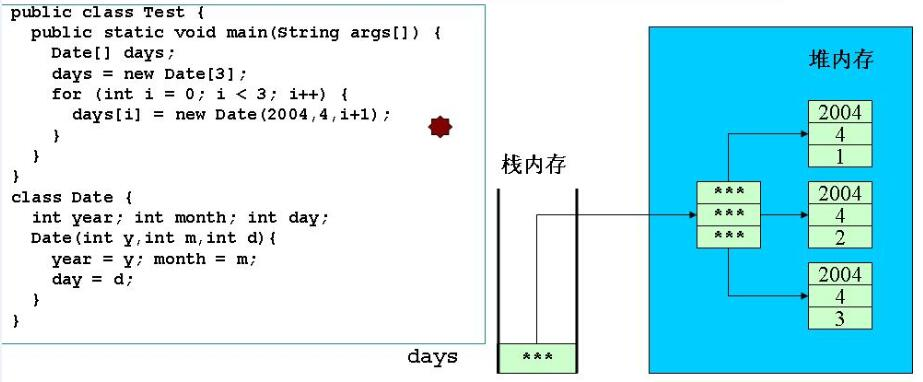3 一维数组的初始化

• 动态初始化：数组定义与为数组元素分配空间和赋值的操作分开进行，先分配空间再赋值。
    public class Test {
public static void main(String[] args){
int[] a;
a = new int;
for (int i=0;i<3;i++){
a[i]=i;
}
Date[] day;
day = new Date;
for (int i=0;i<3;i++){
day[i]= new Date(2016,10,i+1);
}
}
}

class Date{
int year;int month;int day;
Date(int y,int m,int d){
year = y; month = m; day = d;
}
}
• 静态初始化：在定义数组的同时就为数组元素分配空间并赋值。
    public class Test {
public static void main(String[] args){
int[] a = {3,9,8};
Date[] day = {new Date(2016,10,1),
new Date(2016,10,2),new Date(2016,10,3)};
}
}
}

class Date{
int year;int month;int day;
Date(int y,int m,int d){
year = y; month = m; day = d;
}
}

4 数组元素的默认初始化
数组是引用类型，它的元素相当于类的成员变量，因此数组分配空间后，每个元素也被按照成员变量的规则被隐式初始化。

    public class Test {
public static void main(String[] args){
int[] a = new int;
Date[] days = new Date;
System.out.println(a);//0
System.out.println(days);//null
}
}

class Date{
int year;int month;int day;
Date(int y,int m,int d){
year = y; month = m; day = d;
}
}


5 数组元素的引用

• 定义并用运算符new为之分配空间后，才可以引用数组中的每个元素。
• 每个数组都有一个**属性**length指明它的长度，例如a.length的值为数组a的长度（元素个数），不是方法，与String的length()做区别。

选择排序（从小到大）：遍历一次找出最小的数，放到起始位置

int类型排序：

public class NumSort{
public static void main(String[] args){
int[] a = new int[args.length];
for (int i=0;i<args.length;i++){
a[i]=Integer.parseInt(args[i]);
}
print(a);
//selectSort(a);
bubbleSort(a);
print(a);
}
//选择排序
private static void selectSort(int[] a){
int k,temp;
for(int i=0;i<a.length;i++){
k=i;
for(int j=k+1;j<a.length;j++){
if (a[k]>a[j]){
k = j;
}
}
if (k!=i){
temp = a[i];
a[i] = a[k];
a[k] = temp;
}
}
}
//冒泡排序
private static void bubbleSort(int[] a){
int len=a.length;
for(int i=len-1;i>=1;i--){
for (int j=0;j<=i-1;j++){
if (a[j]>a[j+1]){
int temp=a[j];
a[j]=a[j+1];
a[j+1]=temp;
}
}
}
}

private static void print(int[] a){
for(int i=0;i<a.length;i++){
System.out.print(a[i]+" ");
}
System.out.println();
}
}

运行结果：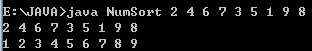引用对象的排序：

public class TestDataSort{
public static void main(String[] args){
Date[] days = new Date;
days = new Date(2006,5,4);
days = new Date(2006,7,4);
days = new Date(2008,5,4);
days = new Date(2004,5,9);
days = new Date(2004,5,4);

bubbleSort(days);
//selectSort(days);

for(int i=0;i<days.length;i++){
//直接打印对象的引用会调用toString
System.out.println(days[i]);
}
}
//冒泡排序
public static Date[] bubbleSort(Date[] a){
int len = a.length;
for (int i=len-1;i>=1;i--){
for(int j=0;j<=i-1;j++){
//如果a[j]>a[j+1]
if (a[j].compare(a[j+1])>0){
Date temp = a[j];
a[j]=a[j+1];
a[j+1]=temp;
}
}
}
return a;
}
//选择排序
public static Date[] selectSort(Date[] a){
int k;
Date temp;
for (int i=0;i<a.length;i++){
k=i;
for (int j=k+1;j<a.length;j++){
if (a[k].compare(a[j])>0){
k=j;
}
}
if (k!=i){
temp=a[i];
a[i]=a[k];
a[k]=temp;
}
}
return a;
}
}

class Date{
int year,month,day;
Date(int y,int m,int d){
year=y;
month=m;
day=d;
}
public int compare(Date date){
return year > date.year ? 1
: year < date.year ? -1
: month > date.month ? 1
: month < date.month ? -1
: day > date.day ? 1
: day < date.day ? -1:0;
}
//重写toString()
public String toString(){
return"Year:Month:Day --"+year+"-"+month+"-"+day;
}
}

运行结果：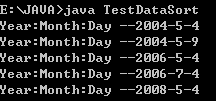二分法： 找到12所在的位置

public class TestSearch{
public static void main(String[] args){
//二分法一定要在排好序的前提下进行
int a[] = {1,3,6,8,9,10,12,18,20,34};
int i = 12;
System.out.println(binarySearch(a,i));
}

public static int search(int[] a,int num){
for (int i=0;i<a.length;i++){
if(a[i] == num)
return i;
}
return -1;
}

public static int binarySearch(int[] a, int num){
if (a.length == 0){
return -1;
}
int startPos =0;
int endPos = a.length-1;
int m=(startPos+endPos)/2;
while (startPos<= endPos){
if (num ==a[m]){
return m;
}
if (num > a[m]){
startPos = m+1;
}
if (num < a[m]){
endPos = m-1;
}
m = (startPos + endPos)/2;
}

return-1;
}

}

6 二维数组
二维数组可以看成以数组为元素的数组，例如：int [][] ={{1,2}，{3,4,5,6}，{7,8,9}};
Java中多维数组的声明和初始化应按从高维到低维（从左到右）的顺序进行，例如：

    int a[][] = new int[];
a = new int;
a = new int;
a = new int;
int t1[][] = new int[];//非法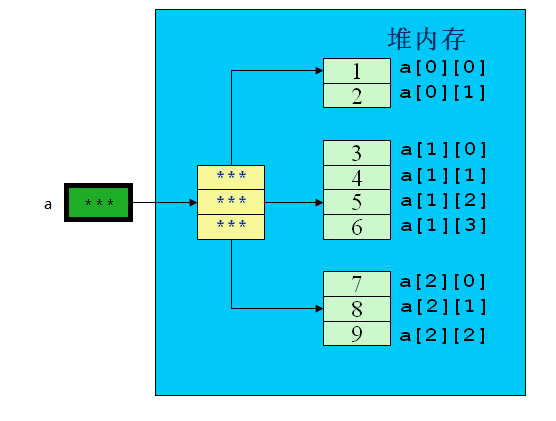静态初始化：int A[][] = {{1,2},{2,3},{3,4,5}};

动态初始化：

    int a[][] = new int;
int b[][] = new int[];
b = new int;
b = new int;
b = new int;

例子程序：

public class TestArray{
public static void main(String[] args){
int a[][]={{1,2},{3,4,5,6},{7,8,9}};
for (int i=0;i<a.length;i++){
for(int j=0;j<a[i].length;j++){
System.out.print("a["+i+"]["+j+"]=" +a[i][j]+" ");
}
System.out.println();
}
}
}

运行结果：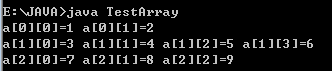String类型的二维数组：

public class TestString{
public static void main(String[] args){
String[][] s = new String[];
s = new String;
s = new String;
s = new String;
for (int i =0;i<s.length;i++){
for(int j=0;j<s[i].length;j++){
s[i][j] =new String("我的位置是："+i+","+j);
}
}
for(int i=0;i<s.length;i++){
for (int j=0;j<s[i].length;j++){
System.out.print(s[i][j]+" ");
}
System.out.println();
}
}
}

运行结果：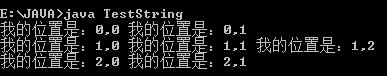数组的拷贝：

• 使用java.lang.System类的静态方法
public static void arraycopy(Object src,int srcPos,Object dest,int destPos,int length);
• 可以用于数组src从第srcPos项元素开始的length个元素拷贝到目标数组从destPos项开始的length个位置
• 如果源数据数目超过目标数组边界会抛出IndexOutOfBoundsException异常。

例子程序：

public class TestArrayCopy{
public static void main(String[] args){
String[] s ={"Microsoft","IBM","Sun","Oracle","Apple"};
String[] sBak = new String;
System.arraycopy(s,0,sBak,0,s.length);

for (int i=0;i<sBak.length;i++){
System.out.print(sBak[i]+" ");
}

System.out.println();
int[][] intArray = {{1,2},{1,2,3},{3,4}};
int[][] intArrayBak = new int[];
System.arraycopy(intArray,0,intArrayBak,0,intArray.length);
intArrayBak = 100;
//虽然没有直接对intArray进行操作，但intArray的值也会发生变化
for(int i=0;i<intArray.length;i++){
for(int j=0;j<intArray[i].length;j++){
System.out.print(intArray[i][j]+" ");
}
System.out.println();
}
}
}

运行结果：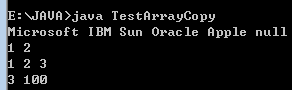展开全文• ## 关于数组的引用和引用的数组

万次阅读 多人点赞 2018-04-27 23:58:36
)array[] array是个数组，其中的元素引用 //应该叫：引用数组 int &amp;array[] //array首先和&amp;结合，所以array是引用引用的对象是数组 //应该叫作数组引用 int (&amp;array) 二、引用...

一、大概区别

就像指针的数组和数组的指针一样耐人寻味

//array首先向右结合，所以这个相当于 (int&)array[] array是个数组，其中的元素是引用

//应该叫：引用的数组

int &array[]


//array首先和&结合，所以array是引用，引用的对象是数组

//应该叫作数组的引用

int (&array)

二、引用的数组

首先，可以明确的说明，引用的数组是不能当函数的参数的。再者要说明，这种方式是非法的

原因：从引用的本质说吧

首先，引用必须被初始化，这没得说，而数组并不能被另一个数组初始化或被另一个数组赋值这是其一，并且其中的每一个引用，你没有办法给他一下子初始化。

再次，引用它不支持传统意义的复制，它不占用新的空间

再再次，他没有意义，它可以用指针数组实现，完全可以代替

int i,j;

int *a = {&i, &j};

a; // point to i

a; // point to j

再再再次，不好实现，因为编译器不知道应该分多大的内存给引用的数组。

三、数组的引用

如果不好理解，可以这样理解（从网上看到的）

int n3 = {2, 4, 6};

int (&rn3) = n3;     //数组的引用


四、数组的引用的应用
void Test(int (&array))
{
cout << "size:" << sizeof(array) << endl;
for (int i = 0; i < sizeof(array)/4; i++)
{
cout << array[i] << " ";
}
}
int main()
{
int  array = {1,2,3,4,5};
Test(array);
return 0;
}

out:

size:20

1 2 3 4 5

展开全文• C语言规定：如果指针变量p已指向数组中的一个元素，则p+1指向同一数组中的下一个元素...*(p+i)或*(a+i)就是p+i或a+i所指向的数组元素，即a[i]。例如，*(p+5)或*(a+5)就是a。指向数组的指针变量也可以带下标，如p[...
• ## C++数组的引用和引用的数组

万次阅读 多人点赞 2018-10-30 15:53:27
)array[] array是个数组，其中的元素引用 //应该叫：引用数组 int &amp;array[] //array首先和&amp;结合，所以array是引用引用的对象是数组 //应该叫作数组引用 int (&amp;array...
•java
• （1）一般情况下数组名都是代表首元素的地址，除了以下两种情况： <1>sizeof（数组名） //注意：（）里仅有数组名，不能有其它的东西 <2>&数组名 （2）sizeof（）函数的作用 sizeof（）计算...
• （1）用字符数组存放一个字符串，可以通过数组名和下标引用字符串中一个字符，也可以通过数组名和格式声明 "%s"输出该字符串。 char string[] ="I Iove china"; printf("%c\n",string); （2）用字符指针变量...
• 数组：Array数组对象是使用单独的变量名来存储一系列的值1、array.slice(start, end)从已有的数组中返回选定的元素，可提取字符串的某个部分，并以新的字符串返回被提取的部分start 必需。规定从何处开始选取。如果...js引用php的数组
• //array首先向右结合，所以这个相当于 (int&)array[] array是个数组，其中的元素引用 //应该叫：引用数组 int &array[] //array首先和&结合，所以array是引用引用的对象是数组 //应该叫作数组引用 int (&...
• 数组变量属引用类型，数组也可以看成是对象，数组中的每个元素相当于该对象的成员变量。  3. 数组中的元素可以是任何数据类型，包括基本类型和引用类型 二、一维数组的声明  1.一维数组的声明方式： type var[ ]...java 数组
• 一维数组和数组元素的地址 前言：定义的数组名可以认为是一个存放地址值的指针变量名，其中的地址是数组第一个元素的地址，也就是数组所占一串连续存储单元的起始地址。重要的是：这个指针变量中的地址值不可改变，...数组 指针
• 引用就是某一变量（目标）的一个别名，对引用的操作与变量直接操作完全一样。 引用的声明方法：类型标识符 &引用名=目标变量名； 【例1】：int a; int &ra=a; //定义引用ra,它是变量a引用，即别名 ...数组引用
• 简单定义、元素的引用：、初始化 定义： 类型名 数组名[整形常量表达式] =...3.在引用数组元素时，数组元素中的下标必须为整数，如果不是整数的话会造成编译错误。 4.一个数组不能同时是使用，数组名中存放的是一个地址
• 数组元素由其所在的位置序号（数据元素的下标）来区分。 数组名与下标的意义：可以用统一的方式来处理数组中的所有元素，从而方便的实现处理一批具有相同性质数据的问题。 注意：数组元素的有序不是指元素大小顺序...
• ## 数组

2019-12-03 23:47:43
数组本身是引用数据类型，而数组中的元素可以是任何数据类型，包括基本数据类型和引用数据类型。 创建数组对象会在内存中开辟一整块连续的空间，而数组名中引用的是这块连续空间的首地址。 数组的长度一旦确定，就不...
• //http://pppboy.blog.163.com/blog/static/3020379620138132495934/一、大概区别就...)array[] array是个数组，其中的元素引用//应该叫：引用数组int &amp;array[]//array首先和&amp;结合，所以array是...
• （这里说的指针的指针不是一个二维数组） 为什么需要使用它们 当我们把一个指针做为参数传一个方法时，其实是把指针的复本传递给了方法，也可以说传递指针是指针的值传递。 如果我们在方法内部修改...
• Java数组、内部排序方法性能比较、排序算法的选择、Arrays工具类的使用java
• 一、大概区别 就像指针的数组和数组的指针一样...//array首先向右结合，所以这个相当于 (int&)array[] array是个数组，其中的元素引用 //应该叫：引用数组 int &array[] //array首先和&结合，所以arr
• 由于[]的优先级，下列先与[]结合，首先确定array为一个数组，然后&与int结合，所以array为一个数组，数组元素为int类型的引用，所以为引用数组。 int &array[]； 而下列中由于括号，array先与&结合，确定array为一...
• 2、指针指向了非法内存,例如定义了一个指针,但是没有指针进行初始化,直接就引用,或者引用里面的元素或者函数,或者没有分配内存就进行释放等,另外,申请内存还要检查是否申请成功,如果没有申请成功也会出现这种情况...数据结构
• Java基础语法之数组的使用及其内存解析【Arrays工具类的使用】java
• 文章目录《C++Primer》第四章-数组和指针-学习笔记(1)-概述指针与数组之间的关系数组数组的定义和初始化显式初始化数组元素特殊的字符数组指针指针是什么取地址操作符指针的定义和初始化指针变量的定义指针可能的...指针 数组...